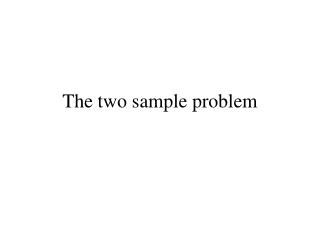DownloadDownload PresentationThe two sample problem

The two sample problem

Télécharger la présentationThe two sample problem

- - - - - - - - - - - - - - - - - - - - - - - - - - - E N D - - - - - - - - - - - - - - - - - - - - - - - - - - -
Presentation Transcript

1. The two sample problem

2. Univariate Inference Let x1, x2, … , xn denote a sample of n from the normal distribution with mean mx and variance s2. Let y1, y2, … , ym denote a sample of n from the normal distribution with mean my and variance s2. Suppose we want to test H0: mx= myvs HA: mx≠ my

3. The appropriate test is the t test: The test statistic: Reject H0 if |t| > ta/2 d.f. = n + m -2

4. The multivariate Test Let denote a sample of n from the p-variate normal distribution with mean vector and covariance matrix S. Let denote a sample of m from the p-variate normal distribution with mean vector and covariance matrix S. Suppose we want to test

5. Hotelling’s T2 statisticfor the two sample problem if H0 is true than has an F distribution with n1= p and n2= n +m – p - 1

6. ThusHotelling’s T2 test We reject

7. Simultaneous inference for the two-sample problem • Hotelling’s T2 statistic can be shown to have been derived by Roy’s Union-Intersection principle

8. Thus

9. Thus

10. Thus Hence

11. Thus form 1 – a simultaneous confidence intervals for

12. Example Annual financial data are collected for firms approximately 2 years prior to bankruptcy and for financially sound firms at about the same point in time. The data on the four variables • x1 = CF/TD = (cash flow)/(total debt), • x2 = NI/TA = (net income)/(Total assets), • x3 = CA/CL = (current assets)/(current liabilties, and • x4 = CA/NS = (current assets)/(net sales) are given in the following table.

13. The data are given in the following table:

14. Hotelling’s T2 test A graphical explanation

15. Hotelling’s T2 statisticfor the two sample problem

16. is the test statistic for testing:

17. Hotelling’s T2 test X2 Popn A Popn B X1

18. Univariate test for X1 X2 Popn A Popn B X1

19. Univariate test for X2 X2 Popn A Popn B X1

20. Univariate test for a1X1+ a2X2 X2 Popn A Popn B X1

21. Mahalanobis distance A graphical explanation

22. Euclidean distance

23. Mahalanobis distance: S, a covariance matrix

24. Hotelling’s T2 statisticfor the two sample problem

25. Case I X2 Popn A Popn B X1

26. Case II X2 Popn A Popn B X1

27. In Case I the Mahalanobis distance between the mean vectors is larger than in Case II, even though the Euclidean distance is smaller. In Case I there is more separation between the two bivariate normal distributions